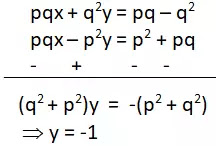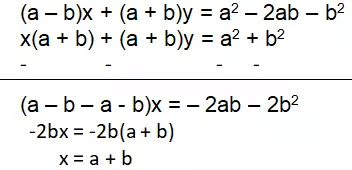# Pair of Linear Equations in Two VariablesExercise – 3.7*

1. The ages of two friends Ani and Biju differ by 3 years. Ani’s father Dharam is twice as old as Ani and Biju is twice as old as his sister Cathy. The ages of Cathy and Dharam differ by 30 years. Find the ages of Ani and Biju.

Let Ani's present age = x years

and present age of Biju = y years

According to Question,

x = y + 3 ……(1)             [If Ani is older than Biju.]

y = x + 3 ……….(2)           [If Biju is older than Ani.]

Ani's father Dharam is twice as old as Ani.

Hence the age of father Dharam = 2x years

Biju's age is twice that of his sister Cathy.

Hence age of Cathy = y/2 years

According to Question,

2x y/2 = 30 ……………..(3)

Substituting the value of x from equation (1),

2(y + 3) – y/2 = 30

4y + 12 – y = 60

3y = 48

y = 16

Hence the present age of Ani is 19 years and the present age of Biju is 16 years.

According to the second condition,

When Biju is older than Ani,

Substituting the value of y from Equation (2) in Equation (3),

2x – (x + 3)/2 = 30

4x – x – 3 = 60

3x = 63

x = 21

Substituting the value of x in equation (2),

y = 21 + 3 = 24

Hence the present age of Ani is 21 years and the present age of Biju is 24 years.

2. One says, “Give me a hundred, friend! I shall then become twice as rich as you”. Tell me what is the other replies, “If you give me ten, I shall be six times as rich as you”. Tell me what is the amount of their (respective) capital? [From the Bijaganita of Bhaskara II]

[ Hint: x + 100 = 2(y – 100), y + 10 = 6(x – 10)]

Let the first friend have Rs.= ₹x

And other friend has Rs. = ₹ y

according to the first condition,

x + 100 = 2(y – 100)

x = 2y – 300 ………….(1)

according to the second condition,

y + 10 = 6(x – 10)

y – 6x = -70

Substituting the value of x from equation (1),

y – 6(2y – 300) = -70

y – 12y + 1800 = -70

-11y = -1870

y = 170

Substituting the value of y in equation (2),

x = 2(170) – 300 = 40

Hence, one friend has Rs 170 and the other has Rs 40.

3. A train covered a certain distance at a uniform speed. If the train would have been 10 km/h faster, it would have taken 2 hours less than the scheduled time. And, if the train were slower by 10 km/h; it would have taken 3 hours more than the scheduled time. Find the distance covered by the train.

Let uniform speed of train = x km/h

and fixed distance = y km

Hence fixed time = y/x hours

According to the first condition,

If the train would have been 10 km/h faster, it would have taken 2 hours less than the scheduled time.

y/(x + 10) = y/x – 2

According to the second condition,

if the train were slower by 10 km/h; it would have taken 3 hours more than the scheduled time.

Substituting the value of y from Equation (1),

x2 = 50x

x = 50

Substituting the value of x in Equation (1),

y = 1/5 (2500 + 500)

y = 1/5 (3000)

y = 600

Hence the distance covered by the train is 600 km.

4. The students of a class are made to stand in rows. If 3 students are extra in a row, there would be 1 row less. If 3 students are less in a row, there would be 2 rows more. Find the number of students in the class.

Let the number of rows = x

and number of students in a row = y

Therefore, total number of students in the class = xy

according to the first condition,

If 3 students are extra in a row, there would be 1 row less.

(x – 1)(y + 3) = xy

xy + 3x – y – 3 = xy

y = 3x – 3 …………………(1)

according to the second condition,

If 3 students are less in a row, there would be 2 rows more.

(x + 2)(y – 3) = xy

xy – 3x + 2y – 6 = xy

-3x + 2y = 6

Substituting the value of y from Equation (1),

-3x + 2(3x – 3) = 6

-3x + 6x – 6 = 6

3x = 12

x = 4

Substituting the value of x in Equation (1),

y = 3×4 – 3 = 9

Total number of students in the class = xy = 4×9 = 36

5. In a ABC, तेज C = 3B = 2(A + B). Find the three angles.

Let, A = x and B = y

Therefore,

C = 3y                       [C = 3B]

3B = 2(A + B)

3y = 2(x + y)

y = 2x …………(1)

In ∆ABC,

A + B + C = 180°

x + y + 3y = 180°

x + 4y = 180°

Substituting the value of y from Equation (1),

x + 4(2x) = 180°

9x = 180°

x = 20°

Substituting the value of x in Equation (1),

y = 2(20°) = 40°

Hence, A = 20°, B = 40°, C = 120°.

6. Draw the graphs of the equations 5x – y = 5 and 3x – y = 3. Determine the co-ordinates of the vertices of the triangle formed by these lines and the y axis.

5x – y = 5 …………..(1)

3x – y = 3 …………..(2)

For each of the three solutions of the equation,

From equation (1),

y = 5x – 5

 x 0 1 2 y -5 0 5

From Eq. (2),

 x 0 1 2 y -3 0 3

The coordinates of the vertices of ∆ABC formed by these lines and y-axis are A(1, 0), B(0, –3) and C(0, –5).

Area of ​​ABC

=1/2 × BC × OA

= ½ × 2 × 1 = 1

Hence, the area of ​​ABC is 1 square unit.

7. Solve the following pair of linear equations:

(i) px + qy = p – q ………….(1)

qx – py = p + q    …………..(2)

Multiplying equation (2) by p and equation (1) by q and subtracting, we getSubstituting the value of y in equation (1),

px + q(-1) = p – q  ⇒  px = p

x = 1

Hence, x = 1 and y = -1.

(ii) ax + by = c ………(1)

bx + ay = 1 + c ……….(2)

Multiplying equation (2) by a and equation (1) by b and subtracting, we get

Substituting the value of y in equation (1),

(iii) x/a – y/b = 0 …………(1)

ax + by = a2 + b2 ………..(2)

From equation (1),

x = ay/b

Substituting the value of x in equation (2),

a(ay/b) + by = a2 + b2

a2y + b2y = a2b + b3

y(a2 + b2) = b(a2 + b2)

y = b

Substituting the value of y in equation (3),

x = (a×b)/b = a

Hence, x = a and y = b.

(iv) (a – b)x + (a + b)y = a2 – 2ab – b2 ……..(1)

(a + b)(x + y) = a2 + b2

x(a + b) + (a + b)y = a2 + b2 ………(2)

Subtracting Equation (2) from Equation (1),Substituting the value of x in equation (1),

(a – b)(a + b) + (a + b)y = a2 – 2ab – b2

a2 – b2 + (a + b)y = a2 – 2ab – b2

(a + b)y = – 2ab

y = -2ab/(a + b)

Hence, x = a + b and y = -2ab/(a + b)

(v) 152x – 378y = -74 …………..(1)

-378x + 152y = -604 …………..(2)

Subtracting Equation (2) from Equation (1),

x – y = 1

x = 1 + y ……………(3)

Substituting the value of x in equation (1),

152(1 + y) – 378y = -74

152 + 152y – 378y = -74

-226y = -226

y = 1

Substituting the value of y in equation (3),

x = 1 + 1 = 2

Hence, x = 2 and y = 1.

8. ABCD is a cyclic quadrilateral (see Figure). Find the angles of the cyclic quadrilateral.

Solution:

The opposite angles of a cyclic quadrilateral are supplementary.

Therefore,

A + C = 180° ……………(1)

B + D = 180° ………………(2)

From Eq. (1),

4y + 20 – 4x = 180

y – x = 40

y = 40 + x ………………(3)

From Eq. (2),

3y – 5 – 7x + 5 = 180

3y – 7x = 180

Substituting the value of y from Equation (3),

3(40 + x) – 7x = 180

120 + 3x – 7x = 180

-4x = 60

x = -15

Substituting the value of x in equation (3),

y = 40 + (-15)

y = 25

Hence, A = 4y + 20 = 4(25) + 20 = 120°

B = 3y – 5 = 3(25) – 5 = 70°

C = -4x = -4(-15) = 60°

D = -7x + 5 = -7(-15) + 5 = 110°

Download Class10 NCRT Pair of Linear Equations in Two Variables Exercise – 3.7* pdf